## Wednesday, October 16, 2013

### A Bayesian Model for a Function Increasing by Chi-Squared Jumps (in Stan)

This post will describe a way I came up with of fitting a function that's constrained to be increasing, using Stan. If you want practical help, standard statistical approaches, or expert research, this isn't the place for you (look up “isotonic regression” or “Bayesian isotonic regression” or David Dunson, whose work Andrew Gelman pointed me to). This is the place for you if you want to read about how I thought about setting up a model, implemented the model in Stan, and created graphics to understand what was going on.

Here's the source for this post, including markdown and and R code, and here's the Stan model it uses.

Many thanks to Naftali Harris for comments and insights on an earlier version.

I recently read a paper that Andrew Gelman wrote back in 1996 about theoretical considerations that can restrict model choice even “in the abence of data with minimal applied context”. As one example, he describes the problem fitting a function $$f(t)$$ that is known to be increasing and where $$f(0)=0$$ and $$f(1)=1$$ are known. We estimate the function given noisy observations of its value at $$(N-1)$$ equally spaced sample points $$\theta_i=f(\frac{i}{N})$$ (for $$i \in \{1,2,3,...,N-1\}$$).

What prior should we use for the $$\{\theta_i\}$$? It might seem like a reasonable “uninformative” prior would make the $$\{\theta_i\}$$ independent and uniform on $$[0,1]$$, with the restriction that non-increasing sets of $$\{\theta_i\}$$ are given zero probability. But this turns out badly! Gelman notes that this prior on the $$\{\theta_i\}$$ is the same as the distribution of the order statistics of a samples of size $$N-1$$ from the uniform distribution on $$[0,1]$$. As the number of points in the discretization increases, the mass of the prior concentrates around a straight line, which will overwhelm the data.

To illustrate, here are some samples from this distribution, for various $$N$$: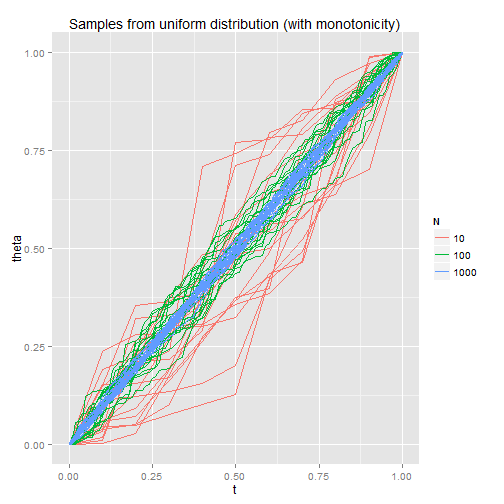This weird! Under the uniform distribution, each (legal) path is equally likely. That's why if we fit this model to noisy data, the maximum likelihood estimate (which is the mode of the posterior) would be reasonable. But it turns out that for large $$k$$, almost all legal paths are basically straight lines, so the bulk of the posterior would be basically a straight line no matter what the data say. It reminds me a bit of statistical mechanics, which is largely about micro-level randomness determining macro-level properties. (I'm no expert, but I recommend this book.)

So what model should we use instead? For my convenience (and perhaps to be more realistic) I'll switch to the case where $$\theta_N$$ (that is, $$f(1)$$) isn't known to be $$1$$. The goal is the have a model which will behave in the same way at each scale of discretization – our results shouldn't differ too much when $$N=10$$ vs. $$N=100$$. To accomplish this, I model the $$\{\theta_i\}$$ as incrementing by jumps drawn from a chi squared distribution. This is nice since 1 jump that's distributed as $$\chi^2(k_0)$$ is then equivalent to $$n$$ independent jumps that are distributed as $$\chi^2(k_0/N)$$. (This means that the function $$f$$ is necessarily discontinuous, which may not be so nice! More on that below.) Then our prior for the function $$f$$ is independent of the scale of the discretization, as long as we scale the parameter correctly:
$\theta_i \sim \theta_{i-1} + s \cdot \chi^2(k_0/N)$
I've added a factor of $$s$$ to the jumps to allow control of the mean and variance of the jumps independently.
I'll assume that we have data $$y_i$$ from a normal distribution centered on $$\theta_i$$ (independent, conditional on $$\theta_i$$). The variance of the $$\{y_i\}$$ should scale with $$N$$, since I'm assuming that if we sampled at a coarser scale, the $$\{y_i\}$$ at a coarser scale would be an average of the $$\{y_i\}$$ at a finer scale (and thus have lower variance):
$y_i \sim N(\theta_i, N \cdot \sigma_y^2)$
I'm giving the hyperparameters $$k_0$$, $$s$$, $$\sigma_y$$ uniform distributions. These aren't meant to be “uninformative” priors at all; just an example (in real life you'd do something reasonable for the problem at hand):
$s \sim U[0,2]$ $k_0 \sim U[10,50]$ $\sigma_y \sim U[0,1]$
This parametrization may not be ideal. Intuitively, maybe how “straight” the line is should be specified independently of how “high” the line goes (here $$k_0$$ affects both)
Here are some samples from my prior for the $$\theta_i$$, conditional on the specified $$k_0$$, $$s$$: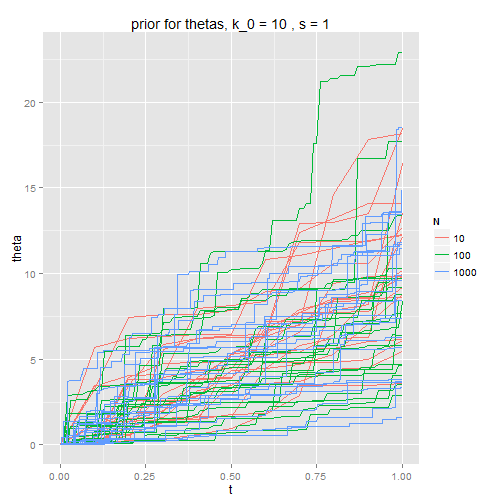As $$k_0$$ gets large, the $$\{\theta_i\}$$ tend toward a straight line.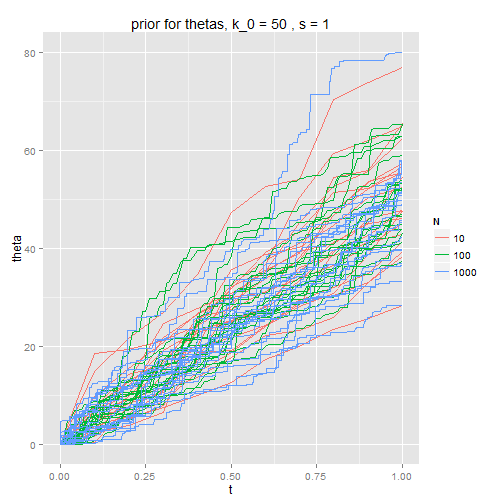Here are samples from my prior for $$\{\theta_i\}$$, sampling from the prior distribution for the hyperparameters $$k_0$$ and $$s$$ (rather than specifying them as above). The shape of the function $$f$$ does not depend on $$N$$ as it did with the naive prior: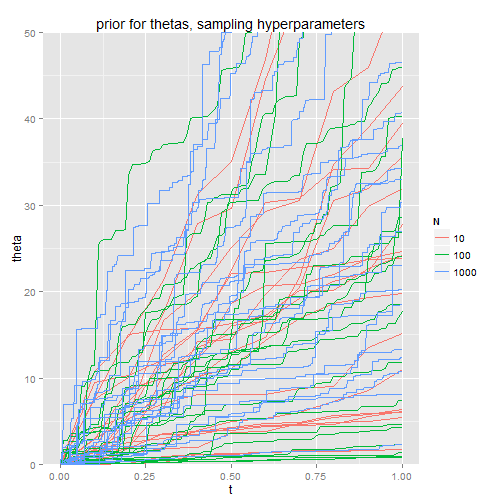I'll illustrate the model by fitting to one set of samples $$\{y_i\}$$, generated by setting with $$k_0=30$$, $$s=1$$, $$\sigma_y=0.5$$, $$N=50$$, and sampling
$\theta_i \sim \theta_{i-1} + s \cdot \chi^2(k_0/N)$
and
$y_i \sim N(\theta_i, N \cdot \sigma_y^2).$
Here are the true $$\{\theta_i\}$$ and the posterior means for $$\{\theta_i\}$$: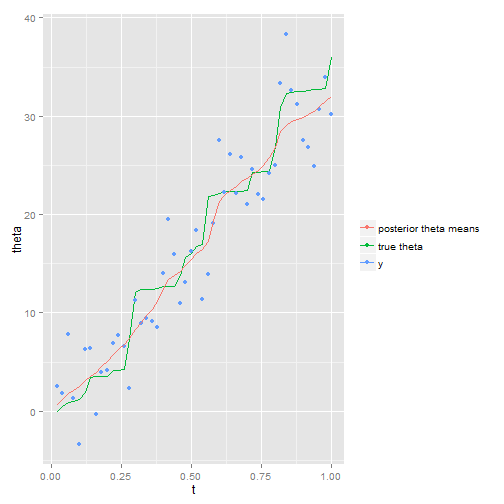Since the posterior means are means, they are quite a lot smoother than the truth. Here are samples from the posterior for $$\{\theta_i\}$$ from each model. You can see that the “shape” of each sample is much more like the shape of the truth: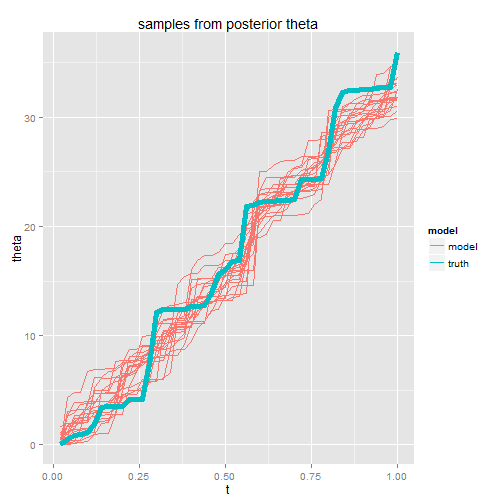The posterior distribution for the hyperparameter $$k_0$$ is very wide – across most of the range of the prior (uniform on $$[10,50]$$), the posterior is not so different from the prior. I think there isn't enough information to fit them well, so the prior will necessarily has a large influence.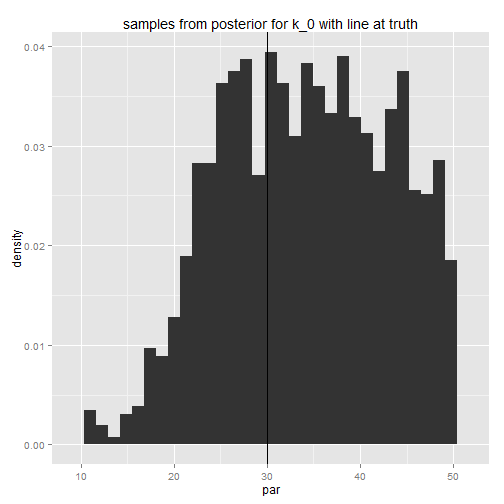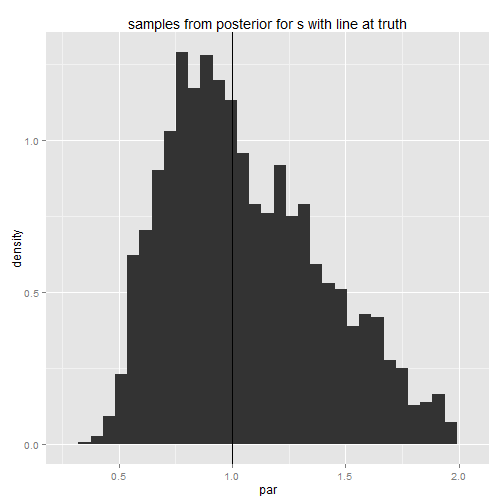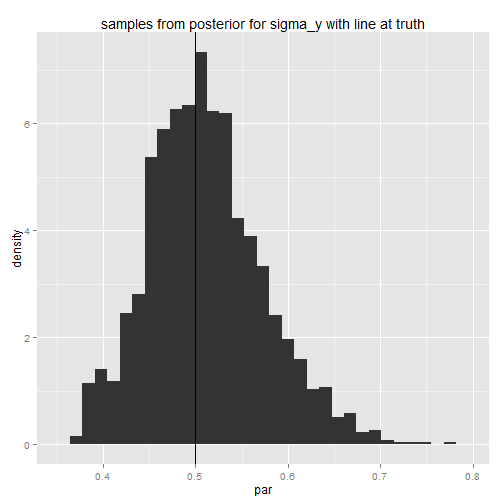Another approach to building a model which would be insensitive to the scale of the discretization would have been to start with a continuous-time, monotone stochastic process on $$[0, 1]$$, and let the joint prior for $$\{\theta_i\}$$ follow from that. That would guarantee up front that the model is reasonable for any degree of discretization. We'd also have the advantage of starting with an understanding of what our process is like in the continuous limit as $$N \rightarrow \infty$$.

For example, I only realized partway through this project that the process I'm describing is discontinuous (in the limit). (Proof: The limit of this process is a Levy process, in that it has stationary, independent increments. The independent increments part is obvious; you even get it in the finite approximations. Levy processes are either Brownian Motion with drift, straight lines, or have discontinuous paths. The limit isn't either of the former two, and so must have discontinuous paths.) A discontinuous model like this might be appropriate for some applications, but not for many. Adding a correlation structure to the jumps would be a reasonable expansion of the model and might be a good way of forcing the paths to be continuous.

Please let me know if you have any comments or suggestions! The flexibility of Stan is very powerful, but that includes the power to fit a bad model. Examining a model's behavior under simulated data is one way to check for things going wrong -- but I still could have messed something up.

1.Neat --- I didn't know about these non-parametric monotonic function models.

Simulated data is totally the way to go to test models. That, and doing the kind of posterior checks you're doing. I just added an intro chapter to the manual for Stan 2.0 that stresses just this point.

It might help others with Stan if you include the model code in the post.

2.Thanks!

I fixed the CSS so the links are more obviously links, and I added a link to the Stan model at the top so that someone who wants that doesn't have to comb through Github for it.

3.Very pedagogical! :) Except for it being a cool model and such, are you planning to use it for some applied problem? I'm sort of wondering when you would want to consider using such a model instead of say a spline based model...

4.No, I don't have any applied plans for this model. This model works for a function which is not continuous and non--decreasing. I think a spline model would be different in both respects, so would work in very different circumstances.

Thanks!

5.Rachel PattersonMay 8, 2018 at 1:07 AM

I guess you could make a chart for Your New Future, if you wish. It's such an amazing skill to get information into numbers.